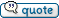Author Message
jrstone54(at)att.net
GuestPosted: Thu Aug 27, 2015 4:38 pm    Post subject: Starting problem- solved```I pasted my original post below describing my starting problem for your reference. I would like to report I have found the solution to my problem. After a very thorough troubleshooting effort throughout the electro air system and my aircraft wiring, I was able to isolate the problem to the magnetic sensor. Of course I had to remove the propeller to get access to the sensor and the crank shaft timing wheel or CSTW. Even though my existing sensor omed-out in the normal range, it apparently was giving a false positive. I replaced the magnetic sensor, propeller and the engine start experience return to normal. Both cold and hot starts are similar to the starts I experienced before removing the impulse magneto. Thanks for your help, hope to meet you guys in the near future. Jim Stone```

 - The Matronics Rocket-List Email Forum - Use the List Feature Navigator to browse the many List utilities available such as the Email Subscriptions page, Archive Search & Download, 7-Day Browse, Chat, FAQ, Photoshare, and much more:http://www.matronics.com/Navigator?Rocket-Listvoltar(at)vx-aviation.com
GuestPosted: Thu Aug 27, 2015 5:23 pm    Post subject: Starting problem- solved```Thanks for the explanation. BTW, I put a blast tube on my pickup. I took one apart to see how they are made and concluded that keeping them cool would help. Vern ======Vernon R. Little | President, Vx Aviation On Aug 27, 2015, at 5:38 PM, Jim Stone <jrstone54(at)att.net (jrstone54(at)att.net)> wrote: [quote] I pasted my original post below describing my starting problem for your reference. I would like to report I have found the solution to my problem. After a very thorough troubleshooting effort throughout the electro air system and my aircraft wiring, I was able to isolate the problem to the magnetic sensor. Of course I had to remove the propeller to get access to the sensor and the crank shaft timing wheel or CSTW. Even though my existing sensor omed-out in the normal range, it apparently was giving a false positive. I replaced the magnetic sensor, propeller and the engine start experience return to normal. Both cold and hot starts are similar to the starts I experienced before removing the impulse magneto. Thanks for your help, hope to meet you guys in the near future. Jim Stone . I'm looking for ideas to improve my start up both cold and hot. I have an IO 540 C4 B5 with a non impulse mag on the upper plugs and ElectroAir Electronic ignition (latest version on the lower plugs), and all aviation plugs. I removed the slick impulse Mag a year ago after the Slick Rep advised me to "get it off that engine before it comes apart and stops your engine". I have been starting with just the ElectroAir and realized it is not my starting technique as much as it is a lack of spark. ElectroAir told me that the system has to measure a pretty fast cranking speed for what seems like a long time before it produces a spark. This cranking pulls the battery voltage down and if the voltage to the ElectroAir controller falls below 11 volts, it no longer produces a spark. Of course I have no indication of no spark other than the obvious no start result. So with some luck the batteries hold up long enough tilI I get the mixture right, if not, recharge and try later. I'm considering the slick start but it requires a different mag from Slick and a coupler which all together cost 2k.. Seems like a big price to pay for better start performance, but I'm considering it. Does anyone have a proven solution? TIA, Jim Stone [url=x-apple-data-detectors://0/1]Louisville Kentucky[/url] HR 11 Quote: D=3D=3D=3D=3D=3D=3D=3D=3D=3D=3D=3D=3D=3D=3D=3D=3D=3D=3D=3D=3D=3D=3D=3D=3D=3D=3D=3D=3D=3D=3D=3D=3D=3D=3D=3D=3D=3D=3D=3D=3D=3D=3D=3D=3D=3D t-List"">http://www.matronics.com/Navigator?Rocket-List D=3D=3D=3D=3D=3D=3D=3D=3D=3D=3D=3D=3D=3D=3D=3D=3D=3D=3D=3D=3D=3D=3D=3D=3D=3D=3D=3D=3D=3D=3D=3D=3D=3D=3D=3D=3D=3D=3D=3D=3D=3D=3D=3D=3D=3D //forums.matronics.com D=3D=3D=3D=3D=3D=3D=3D=3D=3D=3D=3D=3D=3D=3D=3D=3D=3D=3D=3D=3D=3D=3D=3D=3D=3D=3D=3D=3D=3D=3D=3D=3D=3D=3D=3D=3D=3D=3D=3D=3D=3D=3D=3D=3D=3D ot;">http://www.matronics.com/contribution D=3D=3D=3D=3D=3D=3D=3D=3D=3D=3D=3D=3D=3D=3D=3D=3D=3D=3D=3D=3D=3D=3D=3D=3D=3D=3D=3D=3D=3D=3D=3D=3D=3D=3D=3D=3D=3D=3D=3D=3D=3D=3D=3D=3D=3D [b]```

 - The Matronics Rocket-List Email Forum - Use the List Feature Navigator to browse the many List utilities available such as the Email Subscriptions page, Archive Search & Download, 7-Day Browse, Chat, FAQ, Photoshare, and much more:http://www.matronics.com/Navigator?Rocket-Listjrstone54(at)att.net
GuestPosted: Fri Aug 28, 2015 7:55 am    Post subject: Starting problem- solved```Not sure what u saw Lee, there is a newly painted RV10, I have a H-Rocket. Jim On Aug 27, 2015, at 8:58 PM, Lee Logan <leeloganster(at)gmail.com (leeloganster(at)gmail.com)> wrote: [quote]Wasn't that your new paint job on VAF today? If so---WOW! Lee... Quote: [b]```

 - The Matronics Rocket-List Email Forum - Use the List Feature Navigator to browse the many List utilities available such as the Email Subscriptions page, Archive Search & Download, 7-Day Browse, Chat, FAQ, Photoshare, and much more:http://www.matronics.com/Navigator?Rocket-Listsmokyray(at)rocketmail.co
GuestPosted: Fri Aug 28, 2015 11:49 am    Post subject: Starting problem- solved```Jim, Glad you got it solved, knew ya would!!! After all, its not Rocket science...Cya Smokey Sent from my iPhone On Aug 28, 2015, at 10:54, Jim Stone <jrstone54(at)att.net (jrstone54(at)att.net)> wrote: [quote]Not sure what u saw Lee, there is a newly painted RV10, I have a H-Rocket. Jim On Aug 27, 2015, at 8:58 PM, Lee Logan <leeloganster(at)gmail.com (leeloganster(at)gmail.com)> wrote: Quote: Wasn't that your new paint job on VAF today? If so---WOW! Lee... Quote: [b]```

 - The Matronics Rocket-List Email Forum - Use the List Feature Navigator to browse the many List utilities available such as the Email Subscriptions page, Archive Search & Download, 7-Day Browse, Chat, FAQ, Photoshare, and much more:http://www.matronics.com/Navigator?Rocket-ListDisplay posts from previous: All Posts1 Day7 Days2 Weeks1 Month3 Months6 Months1 Year Oldest FirstNewest First
 All times are GMT - 8 Hours Page 1 of 1